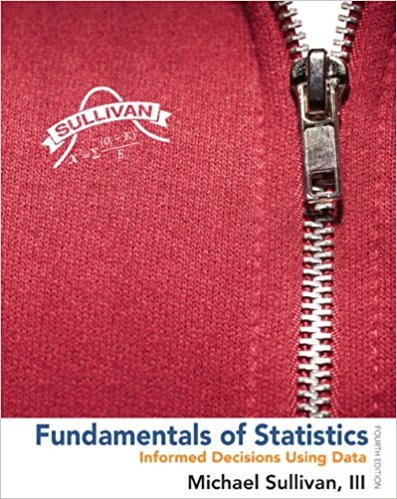×
×

# True or False: A normal score is the expected z-score of aISBN: 9780321838704 30

## Solution for problem 2AYU Chapter Chapter 7.3

Fundamentals of Statistics | 4th Edition

• Textbook Solutions
• 2901 Step-by-step solutions solved by professors and subject experts
• Get 24/7 help from StudySoup virtual teaching assistantsFundamentals of Statistics | 4th Edition

4 5 1 296 Reviews
26
3
Problem 2AYU

True or False: A normal score is the expected z-score of a data value, assuming the distribution of the random variable is normal.

Step-by-Step Solution:
Step 1 of 3

Problem 2AYU

Step1:

True,A normal score is the expected z-score...

Step 2 of 3

Step 3 of 3

##### ISBN: 9780321838704

Unlock Textbook Solution